# RD Sharma Class 9 and Chapter Exponents Of Real Number Maths Solutions

## Maths Class 9 and Chapter Exponents Of Real Number RD Sharma SolutionsFilter
Filters :
CLASSES
•
•
•
•
•
•
•
CHAPTERS
•
• 15 More

# RD Sharma Class 9 Maths Chapter 2 Exponents of Real Numbers

The name ‘exponents’ indicates that this chapter, RD Sharma Class 9 Maths Chapter 2 Exponents of Real Numbers, would deal a lot with calculations that involve raising numbers to exponential powers. This unit again just like the previously learnt ‘Exponents’, deals with certain rules and laws that are applicable when a real number being raised to an exponent has to be solved. Not just that, the interrelation between square roots or cube roots and the exponents can also be figured out through this chapter.

In the current unit, Exponents of Real Numbers, one can see that both rational numbers and irrational numbers are involved here. This unit again is going to be a tough nut to crack and it requires a thorough understanding of the laws of exponents. There are quite a lot of chances that despite having learnt the concepts in schools, the students are prone to get a bit jumbled up when it comes to solving the problems from the unit of Exponents. Such situations make it essential for the students to bank upon study materials such as reference textbooks and solutions guides.

Talking of the reference textbooks, the RD Sharma textbooks have been a popular choice among the CBSE students for many years now. This is despite the fact that there are NCERT books available designed according to the prescribed syllabus of CBSE board. This is because, though the NCERT books tend to cover all that required for the syllabus, the RD Sharma books provide an in-depth explanation of concepts along with a number of worked-out examples and unsolved practise problems.  The RD Sharma books have been entrusted by thousands of students across the country, every year. These books provide enough stuff for not just the school exams, but also all that required to face the engineering entrance exams like IIT JEE Mains and IIT JEE Advanced.

The chapters like the exponents of real numbers are covered sufficiently in the textbooks that will thus lead to the strengthening of the basic skills. The learners can practise well from the exercise problems that are provided in the RD Sharma books and this helps the students to master the chapter. But merely working all the exercise problems would do no good as there needs to be a way through which the students can confirm the accuracy of their solutions. And here arises a need to have a solution guide for the reference textbook through which the students can speed up their learning process and boost their confidence too!

At doubtnut.com, one can find exercise-wise solutions for the RD Sharma textbook worked out and presented in the form of videos. RD Sharma Class 9 Maths Chapter 2 Exponents of Real Numbers are worked out on Doubtnut and the students are welcome to refer to them anytime. Moreover, Doubtnut also provides NCERT solutions for class 9 maths.

Exponents of Real Numbers Class 9 Maths RD Sharma Solutions

The ‘Exponents’, in general words is nothing, but the number of times a number is multiplied by itself. And it is known to all that an exponential number consists of two parts, a base and the exponent. For example, consider the exponential number, 23. Here the number 2 is the base and 3 is the exponent. And 23 is nothing but the number 2 being multiplied three times to itself, that is, 2x2x2 the product of which is 8.

Learning the Exponents of Real Numbers is very important when it comes to studying other subjects such as physics, chemistry, biology and computer science. As it is already known, the exponents are used to represent a number that is really huge through the means of positive exponents, for example, the mass of the earth which is expressed as 5.9*1020. Extremely small numbers can also be represented through the means of negative exponents, for example, 1/106 (or one millionth), can be represented by 10-6 and can be called as micro and represented as ‘μ’.

In the previous years, the students must have studied the chapter, the exponents, and by now have a good understanding of the unit. Now in class 9, through this unit, the exponents of real numbers, is just a continuation of the previously learned unit, the exponents. Through the current unit, one would learn the operations and laws that are involved when solving the exponents. Not just that, the operations of exponents that involve irrational numbers are also learnt here.

Looking at the few laws of exponents that form the core area of this chapter,

Take the base as ‘a’ and ‘m’ and ‘n’ as the exponents.

1. am .an  =am+n
2. (am)n= amn
3. am/ an  = am-n
4. ambm= (ab)m
5. a0          = 1

One can find a lot of problems that make use of these laws appearing in the school exams and the competitive exams. Apart from the application of these laws, this unit also covers the fractional powers. Until now the students only had to deal with positive or negative integers as exponents but now, they would have to work the concept of fractional powers.

Taking an example of a number raised to a fractional number for an exponent, a (1/2). Where a is the base and 1/2 is the exponent. And now, a (1/2) can be expressed as √a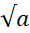. This is called as the square root of ‘a’. And take a (1/3) which is nothing but 3a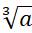, called as the cube root of ‘a’.

So, looking at a few examples using fractional powers,

1. 4(1/2) = √4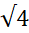= 2 (since 2*2 = 4).
2. 16(1/2) = √16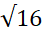= 4 (since 4*4 = 16)
3. 8(1/2) = 38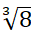= 2 (since 2*2*2 = 8). Similarly, a(1/4) = ∜a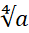, and so on.

These are the main concepts that are covered in this unit in RD Sharma’s textbook. The related video solutions, , on Doubtnut serve as the need of the hour for the students. All the concepts are put across in a simple way so that the students find it easy to understand by themselves through these videos, especially for the ones who are into self-study. Now before going into the study mode, it is also important to know the exact topics covered under the chapter.

## The topics and subtopics of chapter 2 Exponents of Real Numbers

Knowing the topics and subtopics of a given chapter helps the learners be aware of the whole content in a nutshell. This helps them to sort out each topic the way they want to while planning to study. A breakdown of the topics of a particular chapter helps a great deal in designing a suitable study-time table too.

Listing out all the topics and subtopics of chapter 2 Exponents of Real Numbers based on which the class 9 Maths RD Sharma solutions are rolled out on doubtnut.com:

### Laws of exponents

As mentioned above, the problems related to the basic laws of exponents are found in the exercises. It is hence important to understand all these laws well.

### Negative exponents

The concepts of negative exponents and their usage is covered. One can find a lot of problems that involve the usage of negative exponents included here.

### Application of the laws of exponents to the real numbers

Here all concepts of exponents that are applicable to both rational and irrational numbers are included.

### Extended laws of exponents

Some more laws of exponents that are part of the extension of the basic laws, is covered here. These are very helpful when it comes to solving the higher -order problems under the exponents of real numbers. One can find a lot of problems that are of the equation form that demands the application of these laws in order to solve.

Having seen all these topics, it can be noticed that the concepts require higher-order and critical thinking and without using proper materials for learning it would be very difficult for the students to master the concept. Hence, our online tutorial platform takes enough care to break down even the complex problems through easily comprehensible solution methodologies. All the doubts that occur in the learners’ mind can be cleared then and there by just posting a picture of the question on the online portal or the Doubtnut app. The video solutions on doubtnut.com can be paused, played, replayed any number of times as per the learner’s convenience. This makes our tutorials one of its kind and thus has garnered the attention of thousands of students across India.

Share this on :-